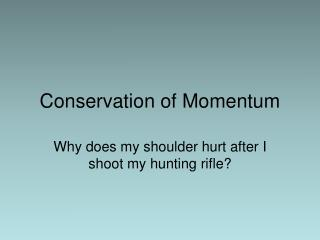DownloadDownload PresentationConservation of Momentum

# Conservation of Momentum

Download Presentation## Conservation of Momentum

- - - - - - - - - - - - - - - - - - - - - - - - - - - E N D - - - - - - - - - - - - - - - - - - - - - - - - - - -
##### Presentation Transcript

1. Conservation of Momentum Why does my shoulder hurt after I shoot my hunting rifle?

2. Conservation of Momentum • pi = pf • In the absence of net external forces acting on the objects, momentum is conserved • Net force provides an impulse, which would result in momentum changing

3. Conservation of Momentum pai = m(v) pbi = m(0) pbf = m(v) paf = m(0)

4. Conservation of Momentum • Momentum is conserved for all objects in the interaction, even if one doesn't stop pai + pbi = paf + pbf

5. Is momentum conserved here? Yes, due to the vector nature of momentum.

6. Is momentum conserved? A B • Initial velocities of both objects is 0. • pai = ma(0) • pbi = mb(0) • Σpi = 0

7. Is momentum conserved? A B • paf = ma(-va) • pbf = mb(vb) • pf = 0 • Σpi = Σpf, so momentum is conserved!! pf = ma(-va) + mb(vb)

8. Why do internal forces result in momentum being conserved? • When Girl A pushes on Girl B, according to Newton’s 3rd Law, Girl B pushes on Girl A • How much? • These forces are equal in magnitude and opposite in direction • The time over which these forces act is exactly the same • Only while the girls are in contact, in this case

9. Why do internal forces result in momentum being conserved? • Impulse is equal in magnitude but opposite in direction • I = (ΣF)(Δt) • Forces are equal and opposite, times are equal • Δp is equal in magnitude, opposite in direction, resulting in Σp = 0!!

10. How does a gun work?

11. How does the gun work? • Only forces are internal (no net external forces are adding impulse to the system) • The momentum of both will add up to zero (bullet is +, gun is -)